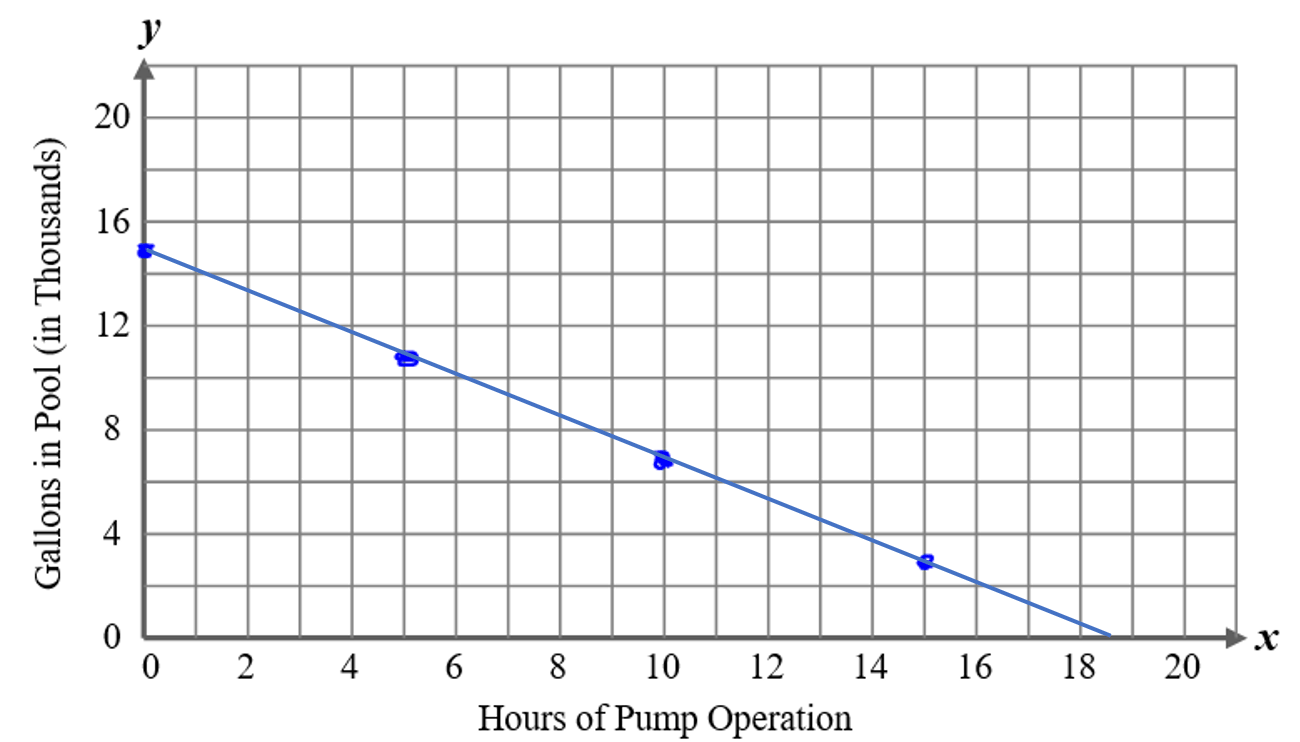# The Situation and Equation:  Solutions

Paul is draining his swimming pool to replace the liner.  Paul’s pool contains $$15,000$$ gallons of water.  He is draining it with a pump that removes $$800$$ gallons per hour.  We can use $$x$$ to represent the number of hours during which the water is pumped and $$y$$ to represent the number of gallons of water remaining in the pool.

1.   Slope: -800 gallons per hour

2.   $$y$$-intercept: ( 0, 15,000 )

3.    $$y=-800+15,000$$

## Table

2.## Graph

3.## Interpreting the Situation

4.  To find the number of hours:

Set the $$y$$-value in the equation, $$y=-800x+15,000$$, equal to zero and solve for $$x$$.

$0=-800x+15,000$$800x=15,000$$\frac{800}{800}x=\frac{15,000}{800}$$x=18.75$

It will take 18 hours and 45 minutes to drain the pool.

5.  The negative $$y$$-values in the table would represent a condition where there would be  negative gallons or that it drained below empty.  Therefore, negative values do not make sense in this context.